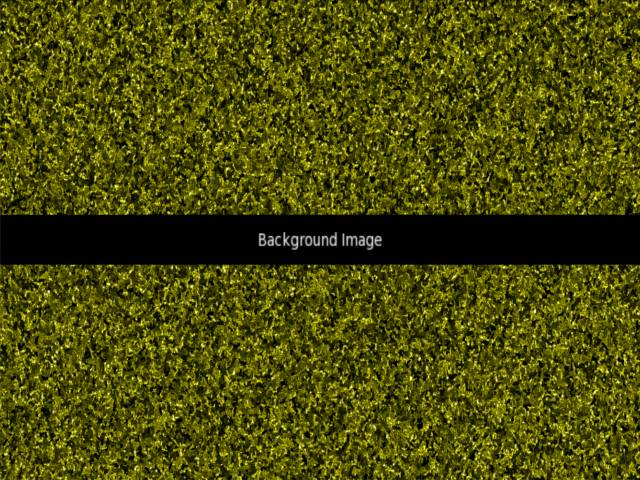-=+=- -=+=- -=+=- -=+=- -=+=- -=+=- -=+=- -=+=- -=+=- -=+=- -=+=- -=+=- -=+=- -=+=- -=+=- -=+=- -=+=- -=+=- -=+=- -=+=- -=+=- -=+=- -=+=- -=+=- -=+=- -=+=- -=+=- -=+=- -=+=- -=+=- (c) WidthPadding Industries 1987 0|577|0 -=+=- -=+=- -=+=- -=+=- -=+=- -=+=- -=+=- -=+=- -=+=- -=+=- -=+=- -=+=- -=+=- -=+=- -=+=- -=+=- -=+=- -=+=- -=+=- -=+=- -=+=- -=+=- -=+=- -=+=- -=+=- -=+=- -=+=- -=+=- -=+=- -=+=-
SoCoder -> Snippet Home -> Generators

PakzCreated : 01 December 2016

System : Other
Language : Other

### Grass like image generator

Modified line function at random pos.

This turned out quite nice imo.

I created a function that draws a line from a to b. It goes step by step and during these steps sometimes it steps in a random direction (x-+ y+-) thus creating not straight lines. You can select different colors and around the line an outline is created with half the color value of the inner part.

I also created code that checks at a random position in the map and counts the higher range colors. If there are enough around that pos then the color of the original position is turned into white(hightlighting)

In high resolution it looks like the picture below. In lower resolutions it looks different.Code is Monkey 2